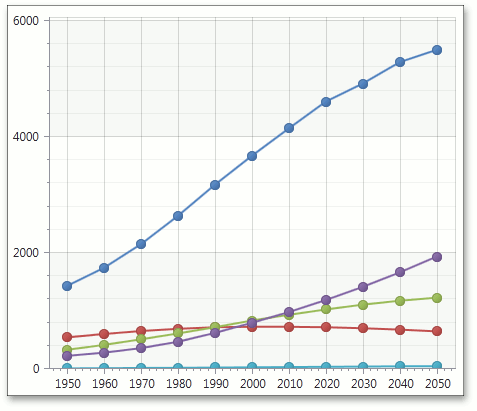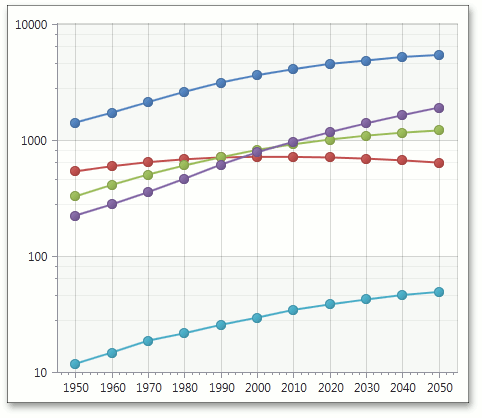20.2 (EAP/Beta)
20.1
19.2
19.1
18.2
18.1
17.2

# Axis.Logarithmic Property

Gets or sets whether the axis should display its numerical values using a logarithmic scale.

Namespace: DevExpress.Xpf.Charts

Assembly: DevExpress.Xpf.Charts.v20.1.dll

## Declaration

``public bool Logarithmic { get; set; }``
``Public Property Logarithmic As Boolean``

## Property Value

Type Description
Boolean

true to display the logarithmic scale; otherwise, false.

## Remarks

If a chart's series has its Series.ArgumentScaleType or Series.ValueScaleType properties set to ScaleType.Numerical, you can use the Logarithmic property to specify whether an axis should use the logarithmic scale to display axis values. When the Logarithmic property is set to true, you can also define a logarithmic base value using Axis.LogarithmicBase.

The axis forms its logarithmic scale as follows. The axis calculates a base axis value - minimum absolute value that is the power of the Axis.LogarithmicBase. These calculations are based on absolute values of data point values that the axis measures. Then, the axis marks positive and negative base values on the axis and next, positive and negative values that are the power of the Axis.LogarithmicBase. Note that if data point values do not contain positive or negative values, the appropriate semi axis is not plotted.

Usually, the Logarithmic property is used when the data range of a chart's series has significant disparity. By enabling this property, the chart's numerical axes display all values using logarithmic equivalents. In short, if the logarithmic base is 10, only 5 uniform axis steps will exist between 10 and 1,000,000.

The following table illustrates how the Logarithmic property works.

Logarithmic = false

Logarithmic = true

LogarithmicBase = 10